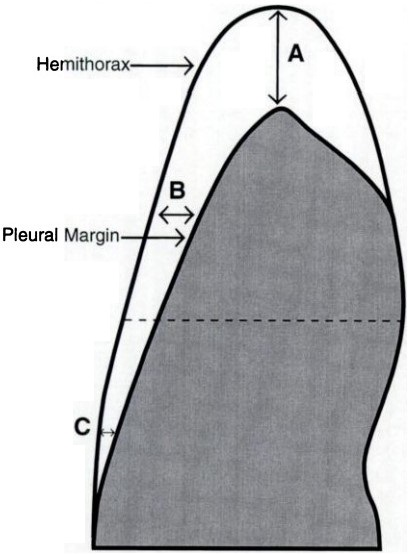# A Step By Step Pneumothorax Volume (PTX) Calculation#Percentage Pneumothorax Size Calculator

This online calculator will calculates the pneumothorax volume percentage.

cm
cm
cm
Step by Step calculation## Formula To Calculate Percentage of Pneumothorax:

PTX, Y = 4.2 + [4.7 x (A+B+C) ] , r = .98, p < .0001.

where,

A is apex to apex lung

B is midpoint upper half

C is midpoint lower half

## Posteroanterior View:###More Calculators

Six Minutes Walk Distance Calculator

NEDOCS Score Calculator

Daily Water Intake Calculator

Temperature Chart & Calculator

Pressure Chart & Calculator

##Age Calculators ►

Horse Age Calculator , Cow Age Calculator , Cat Age Calculator , Dog Age Calculator, Human Age Calculator 100 Days Old Calculator Date Difference Calculator